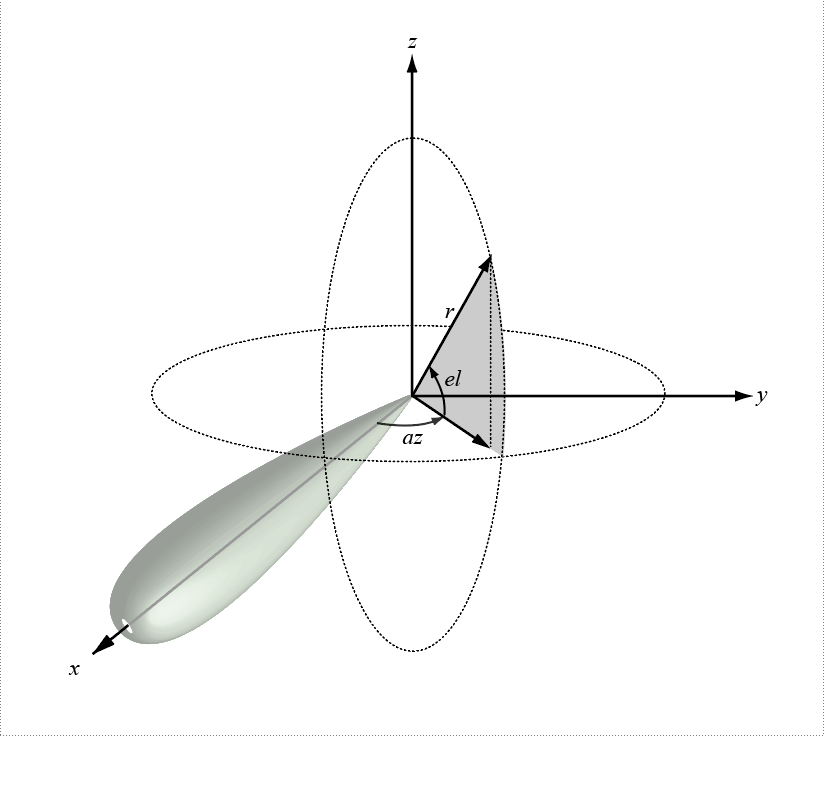# global2localcoord

Convert global to local coordinates

Since R2020a

## Syntax

``lclCoord = global2localcoord(gCoord)``
``lclCoord = global2localcoord(gCoord,option)``
``lclCoord = global2localcoord(___,localOrigin)``
``lclCoord = global2localcoord(___,localAxes)``

## Description

````lclCoord = global2localcoord(gCoord)` converts the global rectangular coordinates `gCoord` to the local rectangular coordinates `lclCoord`. In this syntax, the global coordinate origin is located at (0, 0, 0) and the coordinate axes are the unit vectors in the x, y, and z directions.```
````lclCoord = global2localcoord(gCoord,option)` converts global coordinates to local coordinates using the coordinate transformation type `option`.```

example

````lclCoord = global2localcoord(___,localOrigin)` specifies the origin of the local coordinate system `localOrigin`. Use this syntax with any of the input arguments in previous syntaxes.```

example

````lclCoord = global2localcoord(___,localAxes)` specifies the axes of the local coordinate system `localAxes`. Use this syntax with any of the input arguments in previous syntaxes.```

## Examples

collapse all

Convert the global rectangular coordinates, (0, 1, 0), to local rectangular coordinates. The local coordinate origin is (1, 1, 1).

`lclCoord = global2localcoord([0;1;0],"rr",[1;1;1])`
```lclCoord = 3×1 -1 0 -1 ```

Convert global spherical coordinates to local rectangular coordinates.

`lclCoord = global2localcoord([45;45;50],"sr",[50;50;50])`
```lclCoord = 3×1 -25.0000 -25.0000 -14.6447 ```

Convert two vectors from global to local coordinates using the `global2localcoord` function. Then convert them back from local to global coordinates using the `local2globalcoord` function.

Start with two vectors in global coordinates, (0, 1, 0) and (1, 1, 1). The local coordinate origins are (1, 5, 2) and (-4, 5, 7), respectively.

`gCoord = [0 1;1 1;0 1]`
```gCoord = 3×2 0 1 1 1 0 1 ```
`lclOrig = [1 -4;5 5;2 7];`

Construct two rotation matrices using the rotation functions.

```lclAxes(:,:,1) = rotz(45)*roty(-15); lclAxes(:,:,2) = roty(45)*rotx(35);```

Convert the vectors from global coordinates to local coordinates.

`lclCoord = global2localcoord(gCoord,"rr",lclOrig,lclAxes)`
```lclCoord = 3×2 -3.9327 7.7782 -2.1213 -3.6822 -1.0168 1.7151 ```

Convert the vectors from local coordinates back to global coordinates.

`gCoord1 = local2globalcoord(lclCoord,"rr",lclOrig,lclAxes)`
```gCoord1 = 3×2 -0.0000 1.0000 1.0000 1.0000 0 1.0000 ```

## Input Arguments

collapse all

Global coordinates in rectangular or spherical coordinate form, specified as a 3-by-N matrix. Each column represents one set of global coordinates.

If the coordinates are in rectangular form, each column contains the (x,y,z) components. Units are in meters.

If the coordinates are in spherical form, each column contains (az,el,r) components. az is the azimuth angle in degrees, el is the elevation angle in degrees, and r is the radius in meters.

The origin of the global coordinate system is assumed to be (0, 0, 0). The global system axes are the standard unit basis vectors in three-dimensional space, (1, 0, 0), (0, 1, 0), and (0, 0, 1).

Data Types: `double`

Type of coordinate transformation, specified as a string scalar or character vector. Specify one of the following values.

ValueTransformation
`"rr"` or `'rr'`Global rectangular to local rectangular
`"rs"` or `'rs'`Global rectangular to local spherical
`"sr"` or `'sr'`Global spherical to local rectangular
`"ss"` or `'ss'`Global spherical to local spherical

Data Types: `string` | `char`

Origin of the local coordinate system, specified as a 3-by-N matrix containing the rectangular coordinates of the local coordinate system origin with respect to the global coordinate system. N must match the number of columns of `gCoord`. Each column represents a separate origin. Alternatively, you can specify `localOrigin` as a 3-by-1 vector. If you do so, `localOrigin` expands to a 3-by-N matrix with identical columns.

Data Types: `double`

Axes of the local coordinate system, specified as a 3-by-3-by-N array. Each page contains a 3-by-3 matrix representing axes for a different local coordinate system. The columns of the 3-by-3 matrices specify the local x, y, and z axes in rectangular form with respect to the global coordinate system. Alternatively, you can specify `localAxes` as a single 3-by-3 matrix. If you do so, `localAxes` expands to a 3-by-3-by-N array with identical 3-by-3 matrices. The default value is the identity matrix.

Data Types: `double`

## Output Arguments

collapse all

Local coordinates in rectangular or spherical coordinate form, returned as a 3-by-N matrix. The dimensions of `lclCoord` match the dimensions of `gCoord`.

Data Types: `double`

collapse all

### Azimuth and Elevation Angles

The azimuth angle of a vector is the angle between the x-axis and the orthogonal projection of the vector onto the xy-plane. The angle is positive from the x-axis toward the y-axis. Azimuth angles lie between –180 and 180 degrees. The elevation angle is the angle between the vector and its orthogonal projection onto the xy-plane. The angle is positive toward the positive z-axis from the xy-plane. By default, the boresight direction of an element or array is aligned with the positive x-axis. The boresight direction is the direction of the main lobe of an element or array.

Note

The elevation angle is sometimes defined as the angle a vector makes with the positive z-axis. The MATLAB® and Communications Toolbox™ products do not use this definition.

This figure illustrates the azimuth and elevation angles of a direction vector.Foley, J. D., A. van Dam, S. K. Feiner, and J. F. Hughes. Computer Graphics: Principles and Practice in C, 2nd Ed. Reading, MA: Addison-Wesley, 1995.